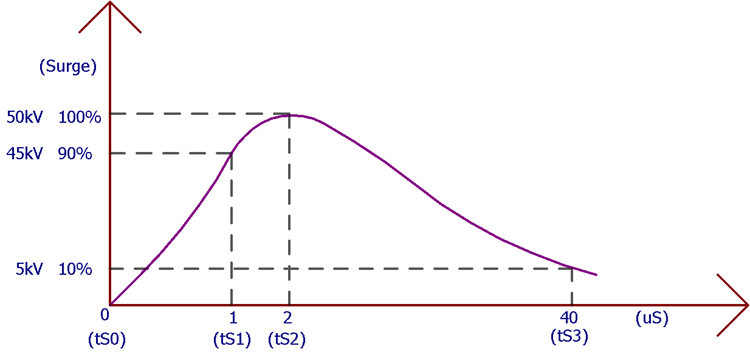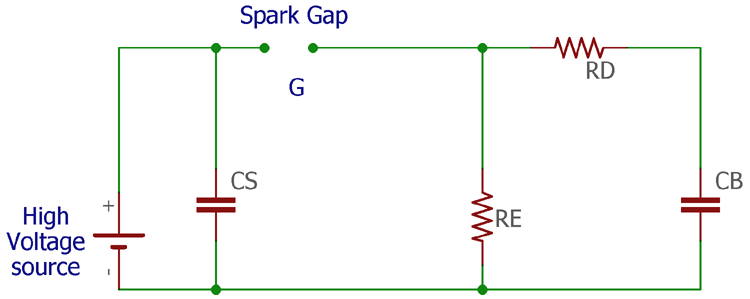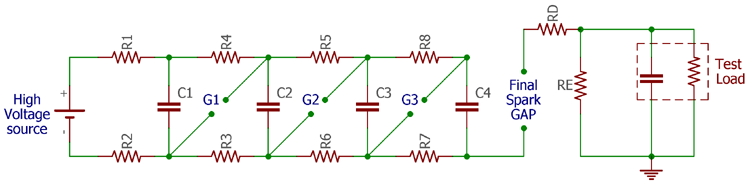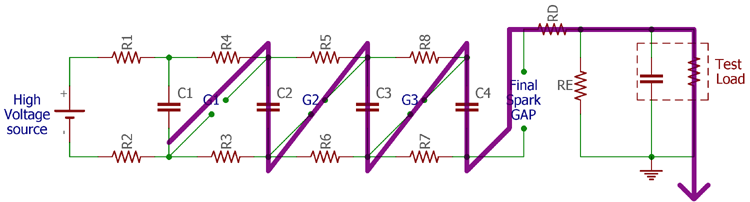Impulse Voltage Generator / Marx Generator – Circuit Diagram, Working Principle and Applications

In electronics, surges are a very critical thing and it is a nightmare for every circuit designer. These surges are commonly referred to as impulse which can be defined as a high voltage, typically in a few kV which exists for a short duration of time. The characteristics of an impulse voltage can be noticed with a High or Low fall time followed by a very high rise time of voltage, Lightning is an example of natural causes which causes Impulse voltage. Since this Impulse voltage can severely damage electrical equipment, it is important to test our devices to work against impulse voltage. This is where we use an Impulse Voltage generator which generates high voltage or current surges in a controlled testing set-up. In this article, we will learn about the working and application of the Impulse Voltage Generator. So, let’s get started.

As told earlier an impulse generator produces this short duration surges with a very high voltage or very high current. Thus, there are two types of impulse generators, impulse voltage generator and impulse current generator. However, in this article, we will discuss impulse voltage generators.

### Impulse Voltage Waveform

To understand the impulse voltage better let’s take a look at the impulse voltage waveform. In the below image, a single peak of high voltage Impulse waveform is shownAs you can see, the wave is getting to its maximum 100 percent peak within 2 uS. This is very fast, but the high voltage is losing its strength with a span of 40uS almost. Therefore, the pulse has a very short or fast rise time whereas a very slow or long fall time. The duration of the pulse is called the wave tail which is defined by the difference between 3rd-time stamp ts3 and ts0.

### Single Stage Impulse Generator

In order to understand the working of an Impulse generator, let stake a look at the circuit diagram of a single-stage impulse generator that is shown belowThe above circuit consists of two capacitors and two resistances. The spark Gap (G) is an electrically isolated gap between two electrodes where electrical sparks happen. A high voltage power source is also shown in the above image. Any impulse generator circuit needs at least one large capacitor that is charged to an appropriate voltage level and then discharged by a load. In the above circuit, the CS is the charging capacitor.  This is a high voltage capacitor typically more than a 2kV rating (depends on the desired output voltage). The capacitor CB is the load capacitance that will discharge the charging capacitor. The resistor and RD and RE control the wave shape.

If the above image observed carefully, we can find that the G or spark gap has no electrical connection. Then how does the load capacitance get the high voltage? Here is the trick and by this one, the above circuit acts as an impulse generator. The capacitor is charged until the capacitor’s charged voltage is enough to cross the spark gap. An electrical impulse generated across the spark gap and high voltage gets transferred from the left electrode terminal to the right electrode terminal of the spark gap and thus making it a connected circuit.

The response time of the circuit can be controlled by varying the distance between two electrodes or changing the capacitors fully charged voltage. The output impulse voltage calculation can be done by calculating the output voltage waveform with

`v(t) = [V0 / CbRd(α – β)] (e – α​t – e – β​t)`

Where,

```α = 1 / RdCb
β = 1 / ReCz```

### Disadvantages of Single Stage Impulse Generator

The major disadvantage of a single-stage impulse generator circuit is the physical size. Depending on the high voltage rating, the components get bigger in size. Also, high impulse voltage generation requires a high DC voltage. Therefore, for a single-stage impulse voltage generator circuit, it gets quite difficult to get optimum efficiency even after using large DC power supplies.

The spheres that are used for the gap connection also required very high in size. The corona that is gets discharged by the impulse voltage generation is very difficult to suppress and reshape. The electrode life gets shorten and requires replacement after a few cycles of repetition.

### Marx generator

Erwin Otto Marx provided a multistage impulse generator circuit in 1924. This circuit is specifically used to generate high impulse voltage from a low voltage power source. The circuit of multiplexed impulse generator or commonly called as Marx circuit can be seen in the below image.The above circuit uses 4 capacitors (there can be n number of capacitors) that are charged by a high voltage source in parallel charging condition by the charge resistors R1 to R8.During the discharge condition the spark gap which was an open circuit during the charging state, acts as a switch and connects a series path through the capacitor bank and generates a very high impulse voltage across the load. The discharge condition is shown in the above image by the purple line. The voltage of the first capacitor needs to be exceeded sufficiently to break down the spark gap and activate the Marx generator circuit.

When this occurs the first spark gap connects two capacitors (C1 and C2). Therefore the voltage across the first capacitor gets double by two voltages of C1 and C2. Subsequently, the third spark gap automatically breaks down because the voltage across the third spark gap is high enough and it starts to add the third capacitor C3 voltage into the stack and this goes on up to the last capacitor. Finally, when the last and final spark gap is reached, the voltage is large enough to break the last spark gap across the load which has a larger gap between the spark plugs.

The final output voltage across the final gap will be nVC (where n is the number of capacitors and VC is the capacitor charged voltage) but this is true in ideal circuits. In real scenarios, the output voltage of the Marx Impulse generator circuit will be much lower than the actual desired value.

However, this last spark point needs to have larger gaps because, without this, the capacitors don’t get into a fully charged condition. Sometimes, the discharge is done intentionally. There are several ways to discharge the capacitor bank in the Marx generator.

Capacitor discharging techniques in Marx Generator:

Pulsing additional Trigger electrode: Pulsing an additional trigger electrode is an effective way to intentionally trigger the Marx generator during fully charge condition or in a special case. The additional trigger electrode is called as Trigatron. There are different shapes and sizes Trigatron available with different specifications.

Ionizing the air in the gap: Ionized air is an effective path that is beneficial to conduct the spark gap. The ionization is done by using a pulsed laser.

Reducing the air pressure inside the gap: The reduction of air pressure is also effective if the spark gap is designed inside a chamber.

### Disadvantages of the Marx Generator

Long charge time: Marx generator uses resistors to charge the capacitor. Thus the charge time gets higher. The capacitor that is closer to the power supply gets charged faster than the others. This is due to the increased distance because of increased resistance between the capacitor and the power supply. This is a major drawback of the Marx generator unit.

Loss of efficiency: Due to the same reason as previously described, as the current flows through the resistors, the efficiency of the Marx generator circuit is low.

The short life span of the spark gap: The repetitive cycle of discharge through the spark gap shortens the lifetime of the electrodes of a spark gap that needs to be replaced from time to time.

The repetition time of charge and discharge cycle: Due to the high charge time, the repetition time of the impulse generator is very slow. This is another major drawback of the Marx generator circuit.

### Application of Impulse Generator Circuit

The major application of the impulse generator circuit is to test high voltage devices. Lightning arresters, Fuses, TVS diodes, different types of surge protectors, etc are tested using the Impulse voltage generator. Not only in the testing field, but the Impulse generator circuit is also an essential instrument that is used in nuclear physics experiments as well as in lasers, fusion and plasma device industries.

The Marx generator is used for the simulation purposes of lightning effects on power-line gear and in aviation industries. It is also used in X-Ray and Z machines. Other uses, such as insulation testing of electronic devices are also tested using impulse generator circuits.

### Get Our Weekly Newsletter!

Subscribe below to receive most popular news, articles and DIY projects from Circuit Digest